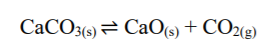The Law of Mass Action and Equilibrium Law↪ From many experimental observations, C.M. Guldberg and P. Waage (Norwegian) in 1863 proposed a generalization in the form of law of action of mass.

↪ An attempt to correlate the rate of a chemical reaction and the concentration of the reactants is known as the Law of Action of Mass.

↪ The law states, "At a constant temperature and pressure, the rate at which substance reacts is proportional to its active mass (or concentration) and the overall rate of a reaction is proportional to the product of active masses (or concentrations) of all the reactants raised to the powers equal to their stoichiometry coefficients in the balanced chemical equation."↪ Active mass here refers to the number of gram moles of the substance dissolved per liter solution which is represented by enclosing the symbol or formula of the substance by square brackets. [ ]

↪ Let us consider a reversible reaction,where a, b, c, d are stoichiometric coefficient of A, B, C and D respectively.

According to law of Mass Action,

Rate of forward reaction ∝ [A]a [B]b →Ⅰ  or
Rate of forward reaction = kf [A]a [B]b

Rate of backward reaction
∝ [C]c [D]dⅡ  or
Rate of backward reaction = kb [C]c [D]d

where [A], [B], [C] and [D] are molar concentration of reactants and products or active mass.
And, kf and kb are rate constant for forward reaction and backward reaction.

At equilibrium,

Rate of forward reaction = Rate of backward reaction [ i.e. rf = rb ]

∴ k[A][B]b = k[C][D]d ﻿
or,where Keq is equilibrium constant.

⁕ Equilibrium Constant

↪ Equilibrium constant can be defined as the ratio between the products of molar concentration of the products to that of the molar concentration of the reactants in the balanced chemical equation.

⁕ Characteristics of Equilibrium Constant

↪ The equilibrium constant has a definite value for every chemical reaction at particular temperature.
↪ It is not affected by the presence of catalyst.
↪ The value of equilibrium constant is independent of initial concentration of reacting species.
↪ The equilibrium constant for the forward reaction is reciprocal that for the backward reaction.

⁕ Significance of Equilibrium Constant

↪ ﻿Higher value of Keq represents the higher concentration of the products at equilibrium and vice versa.

Homogeneous and Heterogeneous equilibrium

⁕ Homogeneous equilibrium

↪ When all reactants and products of a chemical equilibrium are present in the same phases (states), then such type of equilibrium is called homogeneous equilibrium.
For example:⁕ Heterogeneous equilibrium

↪ When the participating chemical species of a chemical equilibrium exists in different phases ( states ), then such type of equilibrium is called heterogeneous equilibrium.
For example: